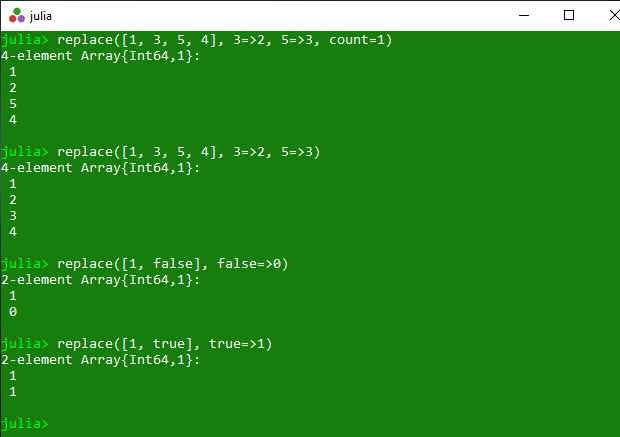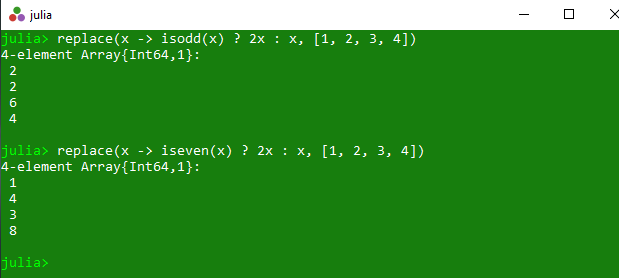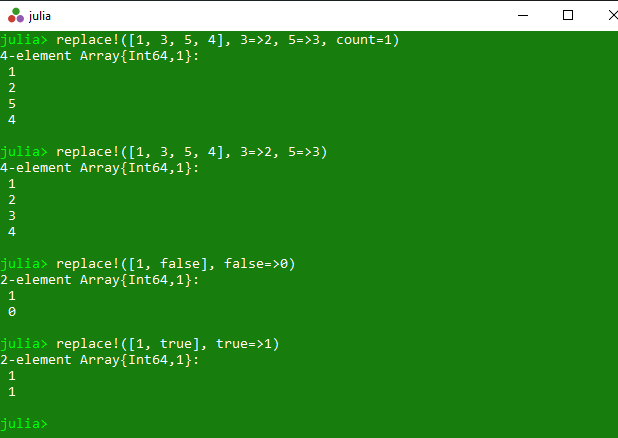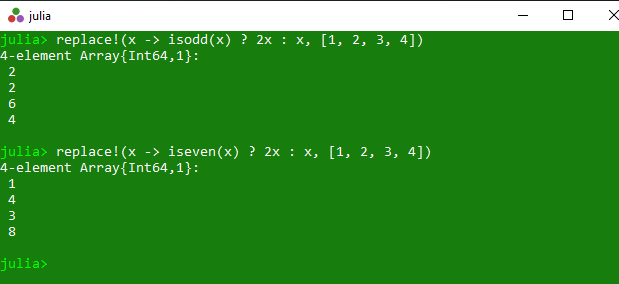Related Articles

# Replacing elements of a collection in Julia – replace() and replace!() Methods

• Last Updated : 01 Apr, 2020

The `replace()` is an inbuilt function in julia which is used to return a copy of the specified array with different types of replacement. These all operations are illustrated with below examples.

Syntax:
replace(A, old_new::Pair…; count::Integer)
or
replace(new::Function, A; count::Integer)

Parameters:

• A: Specified array.
• old_new::Pair: Specified values to get updated.
• count::Integer: This optional count value is used to replace at most count occurrences in total.
• new::Function: Specified set of instruction.

Returns: It returns a copy of the specified array with different types of replacement.

Example 1: This example returns a copy of the specified collection. In this collection old value get replaced with new value and if count is specified, then replace at most count occurrences in total.

 `# Julia program to illustrate ``# the use of replace() method``  ` `# Getting a copy of the specified collection. ``# In this collection old value get replaced ``# with new value and if count is specified, ``# then replace at most count occurrences in total.``println(replace([``1``, ``3``, ``5``, ``4``], ``3` `=``>``2``, ``5` `=``>``3``, count ``=` `1``))``println(replace([``1``, ``3``, ``5``, ``4``], ``3` `=``>``2``, ``5` `=``>``3``))``println(replace([``1``, false], false ``=``>``0``))``println(replace([``1``, true], true ``=``>``1``))`

Output:Example 2: This example returns a copy of a specified array where each value x in the array is replaced by new(x).

 `# Julia program to illustrate ``# the use of replace() method``  ` `# Getting a copy of a specified array ``# where each value x in the array is ``# replaced by new(x).``println(replace(x ``-``> isodd(x) ? ``2x` `: x, [``1``, ``2``, ``3``, ``4``]))``println(replace(x ``-``> iseven(x) ? ``2x` `: x, [``1``, ``2``, ``3``, ``4``]))`

Output:#### replace!()

The `replace!()` is an inbuilt function in julia which is used to return the replaced specified array with different types of replacement. These all operations are illustrated with below examples.

Syntax:
replace!(A, old_new::Pair…; count::Integer)
or
replace!(new::Function, A; count::Integer)

Parameters:

• A: Specified array.
• old_new::Pair: Specified values to get updated.
• count::Integer: This optional count value is used to replace at most count occurrences in total.
• new::Function: Specified set of instruction.

Returns: It returns the replaced array with different types of replacement method.

Example 1: This example returns the replaced collection. In this collection old value get replaced with new value and if count is specified, then replace at most count occurrences in total.

 `# Julia program to illustrate ``# the use of replace !() method``  ` `# Getting the replaced collection``# In this collection old value get replaced ``# with new value and if count is specified, ``# then replace at most count occurrences in total.``println(replace !([``1``, ``3``, ``5``, ``4``], ``3` `=``>``2``, ``5` `=``>``3``, count ``=` `1``))``println(replace !([``1``, ``3``, ``5``, ``4``], ``3` `=``>``2``, ``5` `=``>``3``))``println(replace !([``1``, false], false ``=``>``0``))``println(replace !([``1``, true], true ``=``>``1``))`

Output:Example 2: This example returns the replaced specified array where each value x in the array is replaced by new(x).

 `# Julia program to illustrate ``# the use of replace !() method``  ` `# Getting the replaced specified array ``# where each value x in the array is ``# replaced by new(x).``println(replace !(x ``-``> isodd(x) ? ``2x` `: x, [``1``, ``2``, ``3``, ``4``]))``println(replace !(x ``-``> iseven(x) ? ``2x` `: x, [``1``, ``2``, ``3``, ``4``]))`

Output:My Personal Notes arrow_drop_up